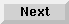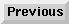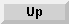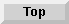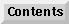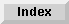7.4 Floating-point numbers

7.4.6 Reference pages

enabled-floating-point-traps Function

Syntax:enabled-floating-point-traps

The functionenabled-floating-point-traps returns a list of the currently enabled conditions that cause interrupt traps on floating-point operations.

You can use the Common Lisp macrosetf to change the list of enabled conditions.

extreme-float-p Function

Syntax:extreme-float-p float

The predicateextreme-float-p identifies extreme floating-point numbers. It returns one of the following values:

• nil

This value indicates that the float argument is a normal or denormalized floating-point number.

• :minus-infinity

This value indicates that the float argument is a negative infinity representation.

• :plus-infinity

This value indicates that the float argument is a positive infinity representation.

• :not-a-number

This value indicates that the float argument is an illegal value, the IEEE NaN condition.

The float argument must be a floating-point number.

integer-decode-float Function

Syntax:integer-decode-float float

The functioninteger-decode-float returns three values:

• The first value is the result of scaling the mantissa of float so that it is an integer. For single-precision numbers, the first value is a fixnum; otherwise, it lies between(expt 2 52) and(expt 2 53).

• The second result is the integer exponent to which the radix must be raised to obtain the value that, when multiplied with the first result, produces the absolute value of the original float value.

• The third result is 1 if float is greater than or equal to zero; otherwise it is -1.

The product of the first result ofinteger-decode-float multiplied by the radix raised to the power of the second result and then multiplied by the third result is exactly equal to the value of the argument.

Returning a fixnum when the first result is a single-precision number is an extension to the Common Lisp behavior for this function.

> (float-precision 0.5)
53

> (integer-decode-float 0.5) 8388608 -24 1

> (= * (expt 2 23)) T

> (let ((*print-base* 16)) (print (integer-decode-float 0.5)) (print (integer-decode-float 0.6)) (print (integer-decode-float (round-to-single-precision 0.6))) nil) 800000 13333333333333 99999A NIL

round-to-single-precision Function

Syntax:round-to-single-precision float

The functionround-to-single-precision rounds a double-precision number (64 bits) down to single-precision format (32 bits); it then reexpands the number to 64-bit size.

The number is stored and prints in 64-bit format.

This function allows you to model the rounding behavior of a machine or implementation that performs 32-bit floating arithmetic.

The default size and rounding mode for Liquid Common Lisp is 64 bits and round-to-nearest, as specified by the IEEE standard.

> pi
3.141592653589793

> (float-precision pi) 53

> (round-to-single-precision pi) 3.1415927410125732

> (float-precision (round-to-single-precision pi)) 53

supported-floating-point-conditions Constant

Syntax:supported-floating-point-conditions

The constantsupported-floating-point-conditions is a list of the floating-point trapping conditions. These conditions conform to the IEEE standard for detecting floating-point exceptions.

The following conditions are supported:

• floating-point-underflow,floating-point-overflow

These conditions occur when the capacity of the floating-point exponent range is exceeded. The underflow condition also occurs with IEEE denormalization and is sometimes called gradual underflow.

• floating-point-inexact

This condition occurs when rounding actually discards some nonzero bits, that is, when the capacity of the mantissa field is exceeded.

• floating-point-invalid-operation

This condition occurs when an operand is not valid for the operation being performed, such as multiplying zero by infinity or dividing zero by zero.

For LCL/SunOS and LCL/Solaris, the conditionfloating-point-invalid-operation represents the union of three lower-level conditions supported by the MC68881 floating-point coprocessor; see the on-line filewizards.doc for more information.

• division-by-zero

This condition occurs when an attempt is made to divide any nonzero number by zero.

The ability to continue past these conditions is hardware specific.

with-floating-point-traps Macro

Syntax:with-floating-point-traps (enable-list disable-list) {form}*

The macrowith-floating-point-traps enables or disables floating-point traps during the execution of the macro body.

The body code specified by the form arguments is executed with a temporary change to the enabled floating-point traps. Those conditions specified in the enable-list argument are turned on, and those in the disable-list argument are turned off. The enable-list and disable-list arguments are evaluated.

> (with-floating-point-traps ('(floating-point-invalid-condition)
supported-floating-point-conditions)
;; Find out how many bits of significance the denormalized
;; format really supports; turn off underflow and loss of
;; of precision traps.
(loop for i from 1
when (zerop (/ least-positive-normalized-single-float
(scale-float 1.0 i)))
return (1- i)))
52

> (with-floating-point-traps ('(floating-point-inexact) ()) (round-to-single-precision pi))

>>Error: A condition of type FLOATING-POINT-INEXACT occurred. EVAL: Required arg 0 (EXPRESSION): (WITH-FLOATING-POINT-TRAPS ((QUOTE #) NIL) (ROUND-TO-SINGLE-PRECISION PI)) :C 0: Use inexact result :A 1: Abort to Lisp Top Level -> :c Use inexact result 3.1415927

;; In the production mode of the compiler, ;; you can get unsafe code. > (compile (defun fast-asin (x) (declare (type (float (0.0) (1.0)) x) (optimize (speed 3) (safety 0))) (asin x))) ;;; Compiling function FAST-ASIN FAST-ASIN

> (with-floating-point-traps (() '(floating-point-OutOfDomain)) (fast-asin 100.0)) #<FLOAT :NOT-A-NUMBER>

> (fast-asin t) ;safety=0 fails to check floatp 5.386404458241357E-169

> ** #<FLOAT :NOT-A-NUMBER>

> (fast-asin *) #<FLOAT :NOT-A-NUMBER>

> (with-floating-point-traps ('(floating-point-NaN) '(floating-point-OutOfDomain)) (fast-asin (fast-asin 100.0)))

#<FLOAT :NOT-A-NUMBER>